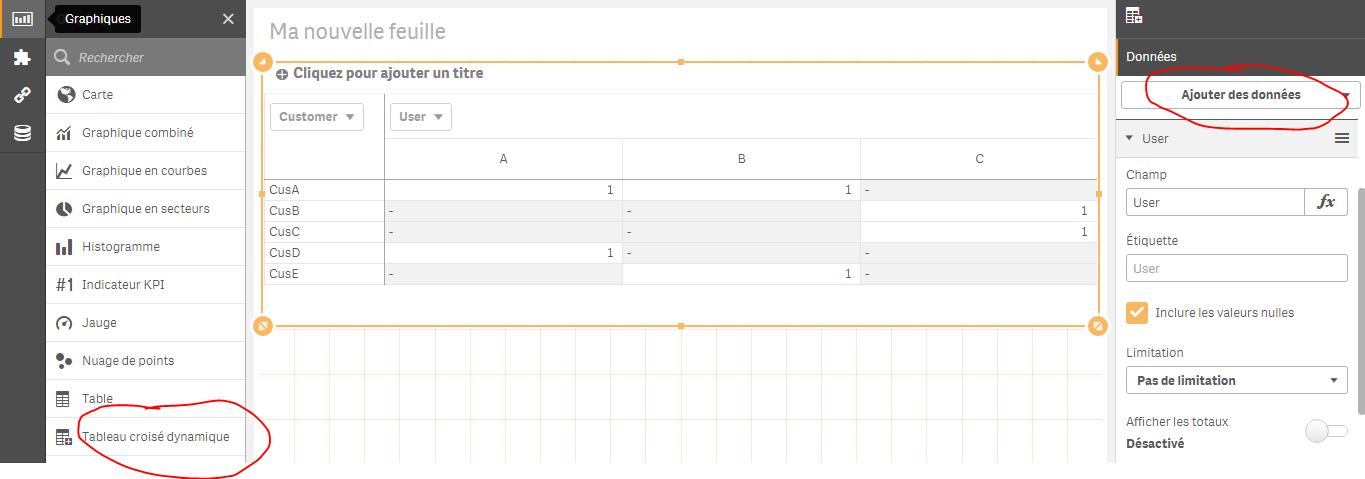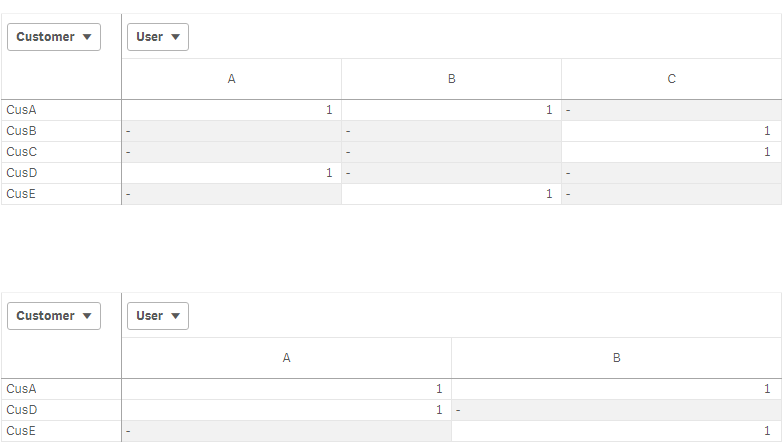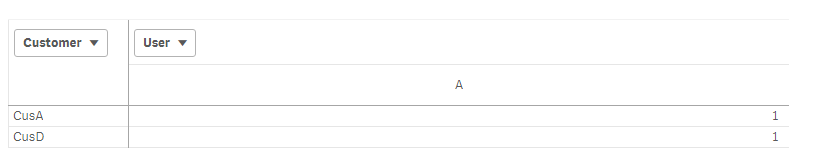# New to Qlik Sense

If you’re new to Qlik Sense, start with this Discussion Board and get up-to-speed quickly.

Announcements
Join “Do More With Qlik” with Mike Tarallo to get a first-hand look at the New Authoring Experience, June 29th: REGISTER HERE
cancel
Showing results for
Did you mean:Not applicable

## SQL statements load script editor(Qlik sense)

Hello, Can someone help me out with understanding, how does one mimic a SQL where clause in the QLIK sense load script editor ?

Also, I am trying to use a sql query to pull some data from multiple tables using left joins followed by a where clause to capture dates in a dynamic range of 1 month going back from today. Any help is appreciated.

1 Solution

Accepted SolutionsPartner

sthing like this:

a,

b,

c;

select a,

b,

c

from souce where a=1;

or

a,

b,

c where a=1 ;

select a,

b,

c

from souce ;

As for left join, as you  probably know, Qlik associate table by column name, if we have table 1 with colum A, B and C and table 2 with columns A , Y and X, Qlik will consider column A as a mapping key.

Now suppose we have:

table 1:

A,

B,

C from source1;

table2:

A,

X,

Y from source 2;

We want to left join table 2 to table 1(table 1 is principle table).

Here's how we proceed

table 1:

A,

B,

C from source1;

left join(table1)

table2:

A,

X,

Y from source 2;

the outcome will be table1 containing ALL columns A,B , C and the joined X AND Y from table 2.

Suppose that we want to only keep columns where A=1?

This is how we alter our script:

table 1:

where A=1;

A,

B,

C from source1;

left join(table1)

table2:

A,

X,

Y from source 2;

I hope that was clear enough?

12 RepliesPartner

sthing like this:

a,

b,

c;

select a,

b,

c

from souce where a=1;

or

a,

b,

c where a=1 ;

select a,

b,

c

from souce ;

As for left join, as you  probably know, Qlik associate table by column name, if we have table 1 with colum A, B and C and table 2 with columns A , Y and X, Qlik will consider column A as a mapping key.

Now suppose we have:

table 1:

A,

B,

C from source1;

table2:

A,

X,

Y from source 2;

We want to left join table 2 to table 1(table 1 is principle table).

Here's how we proceed

table 1:

A,

B,

C from source1;

left join(table1)

table2:

A,

X,

Y from source 2;

the outcome will be table1 containing ALL columns A,B , C and the joined X AND Y from table 2.

Suppose that we want to only keep columns where A=1?

This is how we alter our script:

table 1:

where A=1;

A,

B,

C from source1;

left join(table1)

table2:

A,

X,

Y from source 2;

I hope that was clear enough?Not applicable
Author

Well that was definitely helpful. Thank you.

But to go one step further, how can I write the following statement in qlik ?

SELECT o.*

FROM source.table1  o

where o.date_id >= cast(getdate() - 31 as date)

and o.date_id <= cast(getdate() - 1 as date)Partner

You can write it as it is.

The good thing with Qlik is that you can use its functionalities in the load statement, but also, you can use what your were using within the select statement.

In your case you can do as follow:

SELECT o.*

FROM source.table1  o

where o.date_id >= cast(getdate() - 31 as date)

and o.date_id <= cast(getdate() - 1 as date)Not applicable
Author

Omar, thanks a ton. It all worked. I am assuming all SQL queries will work as it is.

I have a another question digressing from the load script editor. It's regarding the regular script editor.

I have a dataset where users are a dimension and the customer they perform a specific task for is another dimension.

eg:

Let's say the table represents one task only;

User   Customer    Counter(Always 1)

A           Cus A.          1

B           Cus A.          1

C           Cus B.          1

C           Cus C.         1

A           Cus D.         1

B           Cus E.         1

I want to create a table such that, upon selection of a specific user, the table displays the customers the user worked on. Along with these it also shows the other users that touched these customers and other unique customers that were touched by these new users.

For example for I were to select User A from above:

Customer/User     A     B

Cus A.                 1      1

Cus D.                 1      0

Cus E.                 0      1

I hope I was clear enough in trying to explain what I'm looking for. Let me know if you have any suggestions or if I can help explain the above better. Thanks.Partner

You simply use a dynamic table:

As dimension :

Customer

As measure: sum(counter)

then add a column : User:Not applicable
Author

Right, but I do not want user C to be displayed as C and A have no customers in common.

B shows up along with the customers that B touched apart from the ones common to A & B.Partner

Hi again Gautam,

Create the same table

dimension: Customer

Column: User:

Measure: =sum(distinct {<User =P({<Customer={"=Count(Distinct User)>1"}>} User ) >}  Counter)

Result:Hi stalwar1‌, See, when I use the above expression, and click on a user, all the table will turn to null values?

How can I counter this and make it independant of any selection?MVP

May be this

=Sum(distinct {<User =P({<Customer={"=Count({<User>}Distinct User)=2"}>} User ) >}  Counter)

or

=Sum(distinct {<User =P({<Customer={"=Count({1}Distinct User)=2"}>} User ) >}  Counter)Partner

Thank you for the quick answer Sunny but that didn't seem to correct it.

=Sum(distinct {<User =P({<Customer={"=Count({1}Distinct User)=2"}>} User ) >}  Counter)

I aslo tried :

=Sum(distinct {1<User =P({<Customer={"=Count({1}Distinct User)=2"}>} User ) >}  Counter)

But the result is when I select a User, the table will show only the customers of that specific user..